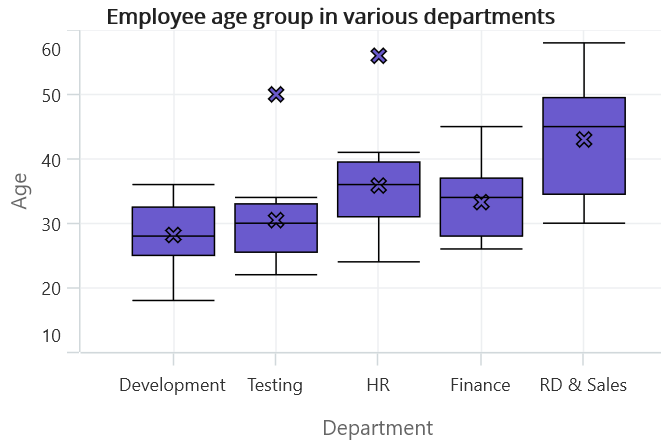# Box and Whisker Chart in .NET MAUI Chart

27 Mar 202313 minutes to read

Box plot chart is used to show the distribution of data within a population. To render a box plot chart, create an instance of BoxAndWhiskerSeries, and add it to the Series collection property of SfCartesianChart.

Box plots are great for comparing the distribution of multiple datasets side by side. By comparing the median, quartiles, and range of the boxes, you can quickly identify differences in the center, spread, and skewness of the distributions. The following code illustrates how to define the series in chart.

NOTE

The Cartesian chart has Series as its default content.

``````<chart:SfCartesianChart>

<chart:SfCartesianChart.XAxes>
<chart:CategoryAxis />
</chart:SfCartesianChart.XAxes>

<chart:SfCartesianChart.YAxes>
<chart:NumericalAxis />
</chart:SfCartesianChart.YAxes>

<chart:BoxAndWhiskerSeries ItemsSource="{Binding BoxWhiskerData}"
XBindingPath="Department"
YBindingPath="Age"/>

</chart:SfCartesianChart>``````
``````SfCartesianChart chart = new SfCartesianChart();
CategoryAxis primaryAxis = new CategoryAxis();
NumericalAxis secondaryAxis = new NumericalAxis();

BoxAndWhiskerSeries series = new BoxAndWhiskerSeries()
{
ItemsSource = new ViewModel().BoxWhiskerData,
XBindingPath = "Department",
YBindingPath = "Age",
};

this.Content = chart;``````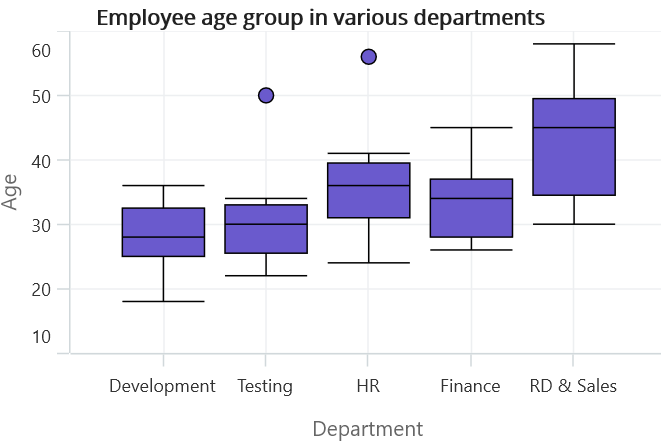## Customize the series Box mode

The series box plotting mode can be changed by using BoxPlotMode property of BoxAndWhiskerSeries. The plotting mode of series can be calculated as follows:

• `Exclusive`- The quartile values are calculated by using the formula (N+1) * P (N count, P percentile) and its index value starts from 1 in the list.

• `Inclusive`- The quartile values are calculated by using the formula (N−1) * P (N count, P percentile) and its index value starts from 0 in the list.

• `Normal`- The quartile values are calculated by splitting the list and getting the median values.

By default, BoxPlotMode value is Exclusive.

## Normal

In Normal mode, the whiskers extend to the minimum and maximum data points within two times the standard deviation of the data. This method assumes that the data is normally distributed. The following code illustrate how to define property BoxPlotMode value as Normal.

``````<chart:SfCartesianChart>

<chart:SfCartesianChart.XAxes>
<chart:CategoryAxis />
</chart:SfCartesianChart.XAxes>

<chart:SfCartesianChart.YAxes>
<chart:NumericalAxis />
</chart:SfCartesianChart.YAxes>

<chart:BoxAndWhiskerSeries ItemsSource="{Binding BoxWhiskerData}"
XBindingPath="Department"
YBindingPath="Age"
BoxPlotMode="Normal"/>

</chart:SfCartesianChart>``````
``````SfCartesianChart chart = new SfCartesianChart();
CategoryAxis primaryAxis = new CategoryAxis();
NumericalAxis secondaryAxis = new NumericalAxis();

BoxAndWhiskerSeries series = new BoxAndWhiskerSeries()
{
ItemsSource = new ViewModel().BoxWhiskerData,
XBindingPath = "Department",
YBindingPath = "Age",
BoxPlotMode = "Normal"/>
};

this.Content = chart;``````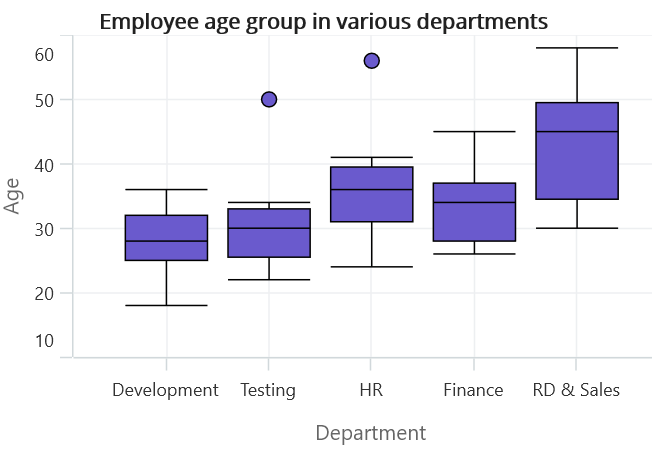## Inclusive

In Inclusive mode, the whiskers extend to the minimum and maximum data points within 1.5 times the IQR. Any points beyond this range are considered outliers. The following code illustrate how to define property BoxPlotMode value as Inclusive.

``````<chart:SfCartesianChart>

<chart:SfCartesianChart.XAxes>
<chart:CategoryAxis />
</chart:SfCartesianChart.XAxes>

<chart:SfCartesianChart.YAxes>
<chart:NumericalAxis />
</chart:SfCartesianChart.YAxes>

<chart:BoxAndWhiskerSeries ItemsSource="{Binding BoxWhiskerData}"
XBindingPath="Department"
YBindingPath="Age"
BoxPlotMode="Inclusive"/>

</chart:SfCartesianChart>``````
``````SfCartesianChart chart = new SfCartesianChart();
CategoryAxis primaryAxis = new CategoryAxis();
NumericalAxis secondaryAxis = new NumericalAxis();

BoxAndWhiskerSeries series = new BoxAndWhiskerSeries()
{
ItemsSource = new ViewModel().BoxWhiskerData,
XBindingPath = "Department",
YBindingPath = "Age",
BoxPlotMode = "Inclusive"/>
};

this.Content = chart;``````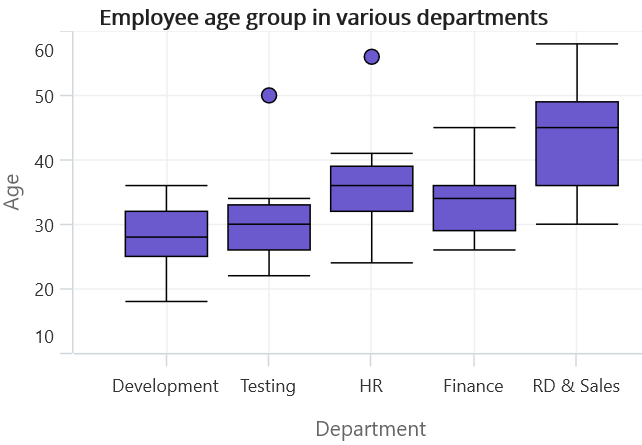## ShowMedian

The Median values of given dataset is viewed by enabling the ShowMedian property of BoxAndWhiskerSeries. By default, the ShowMedian property value is False. The following code illustrates how to enable the ShowMedian property.

``````<chart:SfCartesianChart>

<chart:SfCartesianChart.XAxes>
<chart:CategoryAxis />
</chart:SfCartesianChart.XAxes>

<chart:SfCartesianChart.YAxes>
<chart:NumericalAxis />
</chart:SfCartesianChart.YAxes>

<chart:BoxAndWhiskerSeries ItemsSource="{Binding BoxWhiskerData}"
XBindingPath="Department"
YBindingPath="Age"
ShowMedian="True"/>

</chart:SfCartesianChart>``````
``````SfCartesianChart chart = new SfCartesianChart();
CategoryAxis primaryAxis = new CategoryAxis();
NumericalAxis secondaryAxis = new NumericalAxis();

BoxAndWhiskerSeries series = new BoxAndWhiskerSeries()
{
ItemsSource = new ViewModel().BoxWhiskerData,
XBindingPath = "Department",
YBindingPath = "Age",
ShowMedian = "True"/>
};

this.Content = chart;``````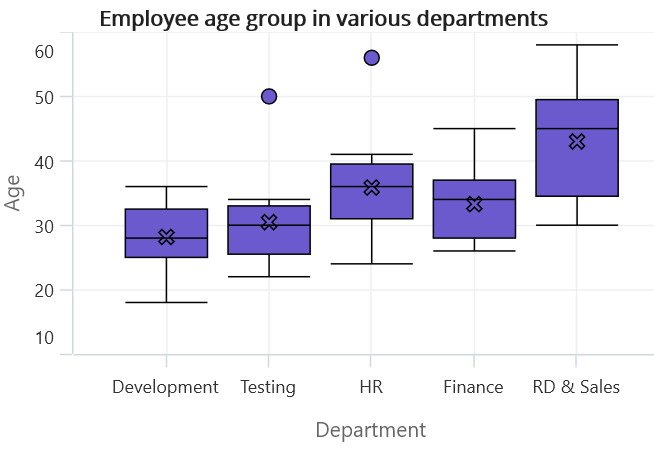NOTE

• The middle number of data points is the median for the odd number of data points.
• The average of the middle two numbers is a median for the even number of data points.

## Outlier

The outlier displays the outlier point that lies either under the lower whisker or above the upper whisker line. It is an observation numerically distant from the rest of the data.

## ShowOutlier

The outlier value in the box plot can be viewed by enabling the ShowOutlier property of BoxAndWhiskerSeries.By default, ShowOutlier value is true. The following code demonstrates how to disable the ShowOutlier property.

``````<chart:SfCartesianChart>

<chart:SfCartesianChart.XAxes>
<chart:CategoryAxis />
</chart:SfCartesianChart.XAxes>

<chart:SfCartesianChart.YAxes>
<chart:NumericalAxis />
</chart:SfCartesianChart.YAxes>

<chart:BoxAndWhiskerSeries ItemsSource="{Binding BoxWhiskerData}"
XBindingPath="Department"
YBindingPath="Age"
ShowOutlier="False"/>

</chart:SfCartesianChart>``````
``````SfCartesianChart chart = new SfCartesianChart();
CategoryAxis primaryAxis = new CategoryAxis();
NumericalAxis secondaryAxis = new NumericalAxis();

BoxAndWhiskerSeries series = new BoxAndWhiskerSeries()
{
ItemsSource = new ViewModel().BoxWhiskerData,
XBindingPath = "Department",
YBindingPath = "Age",
ShowOutlier = "False"/>
};

this.Content = chart;``````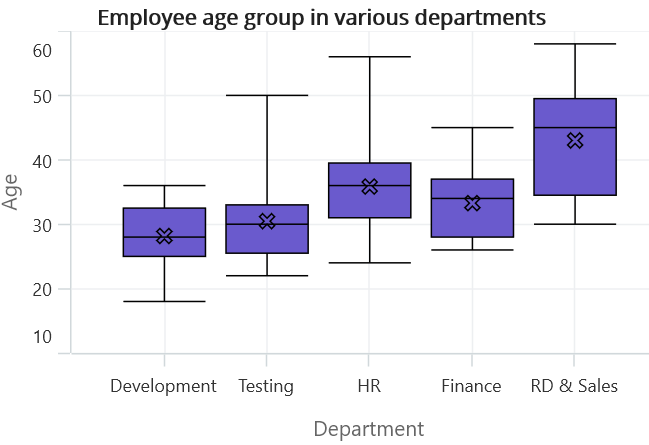## OutlierShapeType

The OutlierShapeType displays the outlier point with different symbols. The available symbols are Cross, Diamond, Circle, Hexagon, InvertedTriangle, Pentagon, Plus, Rectangle and Triangle. By default, OutlierShapeType value is Circle.

The following code shows how to set the OutlierShapeType value as Cross.

``````<chart:SfCartesianChart>

<chart:SfCartesianChart.XAxes>
<chart:CategoryAxis />
</chart:SfCartesianChart.XAxes>

<chart:SfCartesianChart.YAxes>
<chart:NumericalAxis />
</chart:SfCartesianChart.YAxes>

<chart:BoxAndWhiskerSeries ItemsSource="{Binding BoxWhiskerData}"
XBindingPath="Department"
YBindingPath="Age"
OutlierShapeType="Cross"/>

</chart:SfCartesianChart>``````
``````SfCartesianChart chart = new SfCartesianChart();
CategoryAxis primaryAxis = new CategoryAxis();
NumericalAxis secondaryAxis = new NumericalAxis();